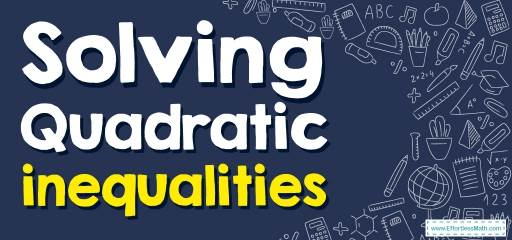# How to Solve Quadratic Inequalities? (+FREE Worksheet!)

Learn how to solve Quadratic Inequalities using similar methods that we use for solving equations.## Step by step guide to solve Solving Quadratic Inequalities

• A quadratic inequality is one that can be written in one of the following standard forms:
$$ax^2+bx+c>0, ax^2+bx+c<0, ax^2+bx+c≥0, ax^2+bx+c≤0$$
• Solving a quadratic inequality is like solving equations. We need to find solutions.

### Solving Quadratic Inequalities – Example 1:

Solve quadratic inequality. $$x^2-6x+8>0$$

Solution:

Factor: $$x^2-6x+8>0→(x-2)(x-4)>0$$
Then the solution could be $$x<2$$ or $$x>4$$.

A Perfect Practice Workbook for Algebra I

### Solving Quadratic Inequalities – Example 2:

Solve quadratic inequality. $$x^2-7x+10≥0$$

Solution:

Factor: $$x^2-7x+10≥0→(x-2)(x-5)≥0$$. $$\space$$ $$2$$ and $$5$$ are the solutions. Now, the solution could be $$x≤2$$ or $$x≥5$$.

### Solving Quadratic Inequalities – Example 3:

Solve quadratic inequality. $$- x^2-5x+6>0$$

Solution:

Factor: $$- x^2-5x+6>0→-(x-1)(x+6)>0$$
Multiply both sides by $$-1: (-(x-1)(x+6))(-1)>0(-1)→(x-1)(x+6)<0$$ Then the solution could be $$-6x$$ and $$x>1$$. Choose a value between $$-1$$ and $$6$$ and check. Let’s try $$0$$. Then: $$- 0^2-5(0)+6>0→6>0$$. This is true! So, the answer is: $$-6<x<1$$

### Solving Quadratic Inequalities – Example 4:

Solve quadratic inequality. $$x^2-3x-10≥0$$

Solution:

Factor: $$x^2-3x-10≥0→(x+2)(x-5)≥0. -2$$ and $$5$$ are the solutions. Now, the solution could be $$x≤-2$$ or $$x≥5$$.

## Exercises for Solving Quadratic Inequalities

• $$\color{blue}{x^2+7x+10<0}$$
• $$\color{blue}{ x^2+9x+20>0}$$
• $$\color{blue}{x^2-8x+16>0}$$
• $$\color{blue}{ x^2-8x+12≤0}$$
• $$\color{blue}{ x^2-11x+30≤0}$$
• $$\color{blue}{ x^2-12x+27≥0}$$

• $$\color{blue}{-5<x<-2}$$
• $$\color{blue}{x<-5 \ or \ x>-4}$$
• $$\color{blue}{x<4 \ or \ x>4}$$
• $$\color{blue}{2≤x≤6}$$
• $$\color{blue}{5≤x≤6}$$
• $$\color{blue}{x≤3 \ or \ x≥9}$$

The Absolute Best Books to Ace Algebra

### What people say about "How to Solve Quadratic Inequalities? (+FREE Worksheet!) - Effortless Math: We Help Students Learn to LOVE Mathematics"?

No one replied yet.

X
45% OFF

Limited time only!

Save Over 45%

SAVE $40 It was$89.99 now it is \$49.99